# Repeated limit

A limit of a function of several variables in which the passage to the limit is performed successively in the different variables. Let, for example, a functionof two variablesandbe defined on a set of the form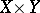,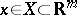,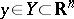, and letandbe limit points of the setsand, respectively, or the symbol(if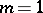or,and, respectively,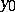may be infinities with signs: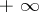,). If for any fixedthe limit(1)

exists, and for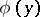the limit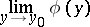exists, then this limit is called the repeated limit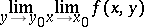(2)

of the functionat the point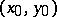. Similarly one defines the repeated limit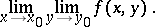(3)

If the (finite or infinite) double limit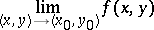(4)

exists, and if for any fixed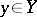the finite limit (1) exists, then the repeated limit (2) also exists, and it is equal to the double limit (4).

If for eachthe finite limit (1) exists, for eachthe finite limit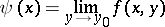exists, and for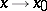the function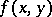tends to a limit functionuniformly in, then both the repeated limits (2) and (3) exist and are equal to one another.

If the setsandare sets of integers, then the functionis called a double sequence, and the values of the argument are written as subscripts:and the repeated limits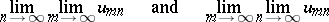are called the repeated limits of the double sequence.

The concept of a repeated limit has been generalized to the case whereandand the set of values of the functionare subsets of certain topological spaces.

How to Cite This Entry:
Repeated limit. L.D. Kudryavtsev (originator), Encyclopedia of Mathematics. URL: http://encyclopediaofmath.org/index.php?title=Repeated_limit&oldid=17217
This text originally appeared in Encyclopedia of Mathematics - ISBN 1402006098